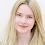## Tuesday, November 11, 2014

### dictzip

def dictzip(*dicts):
return dict([(k, tuple([d[k] for d in dicts]))
for k in set(dicts).intersection(*dicts[1:])])

zip() is a very handy function that takes a series of iterables and returns a list of tuples of the nth elements of each iterable:

>>> zip(['a', 'b', 'c'], [1, 2, 3])
[('a', 1), ('b', 2), ('c', 3)]

dictzip() takes an iterable of dictionaries and returns a dictionary of tuples of the elements of each dict on the same key:

>>> dictzip( {'a': 1}, {'a': 'cat'} )
{'a': (1, 'cat')}

This version is exclusive -- only keys that are in all dictionaries are output.  A simple variant would be to substitute None for missing keys.

def dictzip(*dicts):
return dict([(k, tuple([d.get(k) for d in dicts]))
for k in set(dicts).union(*dicts[1:])])

1.